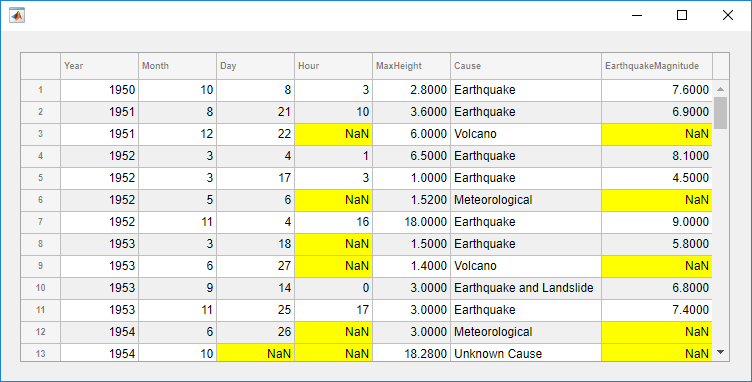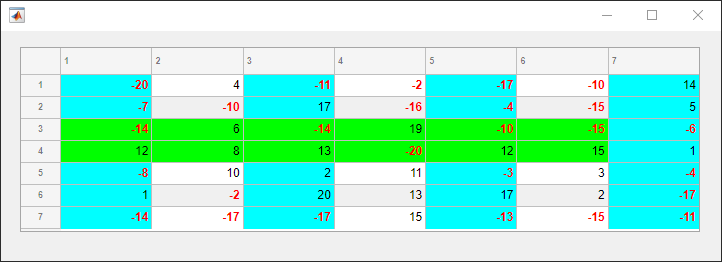## 语法

``addStyle(uit,s)``
``addStyle(uit,s,target,targetIndex)``

## 说明

``addStyle(uit,s)` 将使用 `uistyle` 函数创建的样式添加到指定的表 UI 组件中。该样式将应用于整个表。该表必须是使用 `uifigure` 函数创建的图窗的父级，或其子容器之一的父级。`

``addStyle(uit,s,target,targetIndex)` 将样式添加到一个特定的行、列或单元格中。例如，`addStyle(uit,s,'column',3)` 将样式添加到指定表的第三列。`

## 示例

```fig = uifigure; fig.Position = [500 500 520 200]; uit = uitable(fig); uit.Data = rand(5); uit.Position = [20 30 480 135];``````s = uistyle('FontAngle','italic','FontColor','r'); addStyle(uit,s)``````tdata = readtable('tsunamis.xlsx'); vars = {'Year','Month','Day','Hour', ... 'MaxHeight','Cause','EarthquakeMagnitude'}; tdata = tdata(1:100,vars); fig = uifigure('Position',[500 500 750 350]); uit = uitable(fig); uit.Position = [20 20 710 310]; uit.Data = tdata; uit.RowName = 'numbered';```

```styleIndices = ismissing(tdata); [row,col] = find(styleIndices); s = uistyle('BackgroundColor','yellow'); addStyle(uit,s,'cell',[row,col]);``````fig = uifigure; fig.Position = [500 500 720 230]; uit = uitable(fig); uit.Data = randi([-20,20],7); uit.Position = [20 30 680 185]; [row,col] = find(uit.Data < 0);```

```s1 = uistyle; s1.BackgroundColor = 'cyan'; addStyle(uit,s1,'column',[1 3 5]) s2 = uistyle; s2.FontColor = 'red'; s2.FontWeight = 'bold'; addStyle(uit,s2,'cell',[row,col]) s3 = uistyle; s3.BackgroundColor = 'green'; addStyle(uit,s3,'row',[3 4]) addStyle(uit,s1,'column',7)```## 输入参数

`'row'`

`4`

`[3 8 9 12]`

`'column'`

`3`

`[1 2 7 14]`

`'cell'`

`[2 4;5 9;13 27]`

`'table'`

`''`

`'column'`

`"Torque"`

`["Torque" "Mass"]`

`'Revenue'`

`{'Year','Expenses','Revenue'}`

## 提示

• 要查看已添加到表的样式的列表，请查询 `StyleConfigurations` 属性的值。